基于卷积神经网络的品牌LOGO识别原理与代码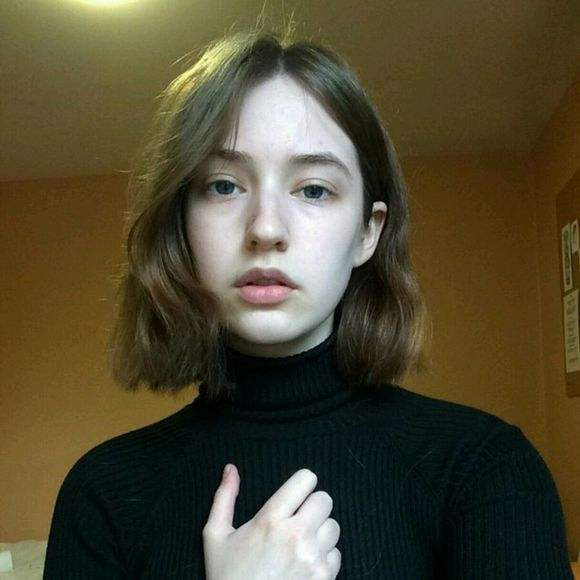ezpod 2019-08-16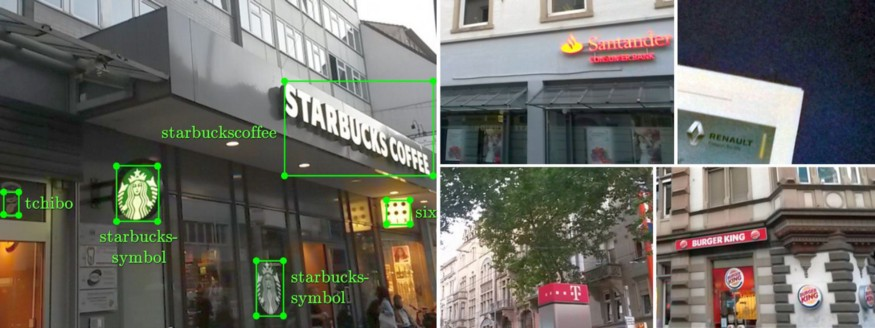1、数据集选择与预处理

from sklearn.datasets import load_files
from keras.utils import np_utils
import numpy

#load files from LogosInTheWild directory with logo categories given by their subfolder name
logo_files = numpy.array(data['filenames'])
jpg_indices = [index for index, name in enumerate(logo_files) if 'jpg'==name.split('.')[-1]]
logo_targets = np_utils.to_categorical(numpy.array(data['target']), max(data['target']+1))
all_files, all_targets = logo_files[jpg_indices], logo_targets[jpg_indices]

from sklearn.model_selection import train_test_split

#split into 80% training+validation files+labels, and 20 % test files+labels
train_and_val_files, test_files, train_and_val_targets, test_targets
= train_test_split(all_files, all_targets, test_size=0.2)
#further split into 80% training files+labels, and 20 % validation files+labels
train_files, val_files, train_targets, val_targets
= train_test_split(train_and_val_files, train_and_val_targets, test_size=0.2)

#create a checkpointer to save the CNN model with the best weight parameters
from keras.callbacks import ModelCheckpoint
checkpointer = ModelCheckpoint(filepath='saved_models/weights.best.from_scratch.hdf5',
save_best_only=True)

from keras.preprocessing import image
from PIL import ImageFile

def path_to_tensor(img_path):
# loads RGB image as PIL format with 224x224 pixels
img = image.load_img(img_path, target_size=(224, 224))
# convert to 3D tensor with shape (224, 224, 3) with 3 RGB channels
x = image.img_to_array(img)
# convert 3D tensor to 4D tensor with shape (1, 224, 224, 3) and return it
return numpy.expand_dims(x, axis=0)

def paths_to_tensor(img_paths):
list_of_tensors = [path_to_tensor(img_path) for img_path in img_paths]
#stack the (1,224,224,3) 4D tensor arrays to a (# images,224,224,3) 4D tensor
return numpy.vstack(list_of_tensors)

#create 4D tensors and rescale each pixel by dividing by RGB max value 255
train_tensors = paths_to_tensor(train_files).astype('float32')/255
val_tensors = paths_to_tensor(val_files).astype('float32')/255
test_tensors = paths_to_tensor(test_files).astype('float32')/255

2、CNN识别店铺LOG的原理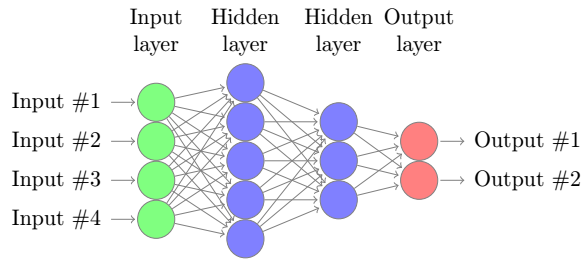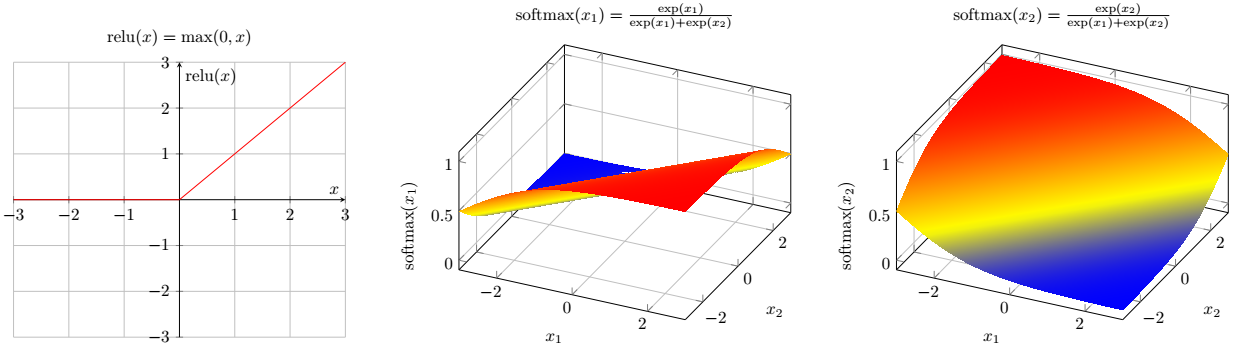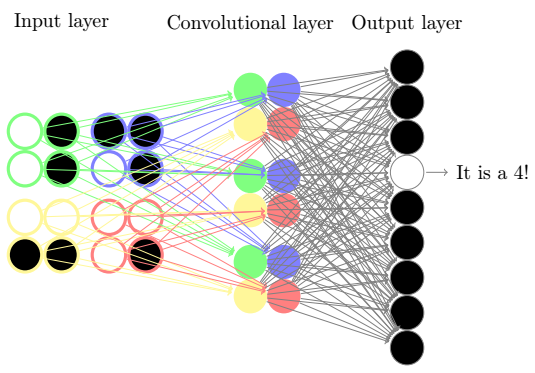3、训练Keras卷积神经网络LOGO识别器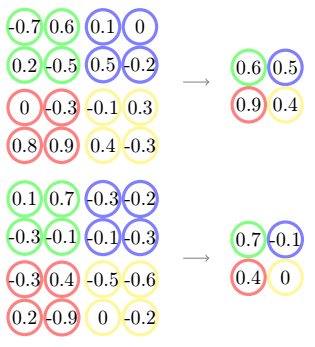-0.1–0.2–0.4–0.1 = - 0.2，可以看到结果特征图中只包含一个节点，因此全局平均池化层可以显著降低特征图的维度。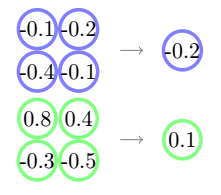from keras.layers import Conv2D, MaxPooling2D, GlobalAveragePooling2D, Dropout, Dense
from keras.models import Sequential

cnn_model = Sequential()

#after the input layer, add the first convolutional layer with 32 2x2-filters
cnn_model.add(Conv2D (kernel_size = (2,2), filters = 32,
input_shape=train_tensors.shape[1:], activation='relu'))
#add a max pooling layer with a 2x2 pooling window
#add the second convolutional layer with 64 2x2-filters
cnn_model.add(Conv2D(kernel_size = 2, filters = 64, activation='relu'))
#add the third convolutional layer with 128 2x2-filters
cnn_model.add(Conv2D(kernel_size = 2, filters = 128, activation='relu'))
#add a dropout layer so that each node has a chance of 20% to be dropped when training
#add a global average pooling layer
#add the final fully connected output layer with 109 node for all 109 logo classes
cnn_model.add(Dense(109, activation = 'softmax'))

cnn_model.summary()

#Compile the model

#Train the model
H = cnn_model.fit(train_tensors, train_targets, epochs=100, verbose=1
validation_data=(val_tensors, val_targets), callbacks=[checkpointer])

#After training, load the model with the best validation loss

4、模型评估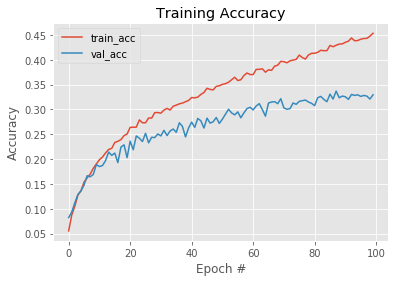5、使用训练好的模型预测LOGO分类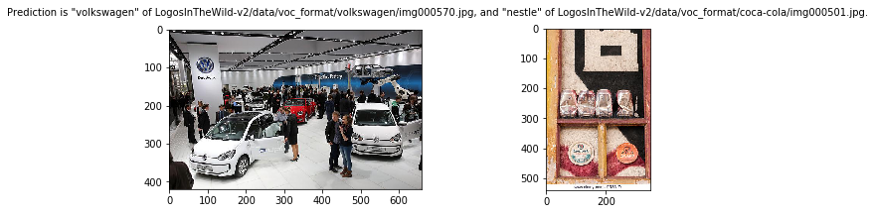corcosa
14056人浏览
2019-10-08

Jarvis-拍立淘里面的深度学习引擎
3542人浏览
2017-11-03 10:58:48

1083人浏览
2017-09-01 13:18:00

576人浏览
2017-08-02 11:12:00
2016年，你给阿里云打几分？
6320人浏览
2016-12-30 18:42:14

35705人浏览
2017-10-04 23:31:42
AI学习路线
3761人浏览
2019-03-12 09:02:11
0
0
318